DAY 5
0
AI & Data

-----阿峻 20190921

# Torchvision

## 簡介以及安裝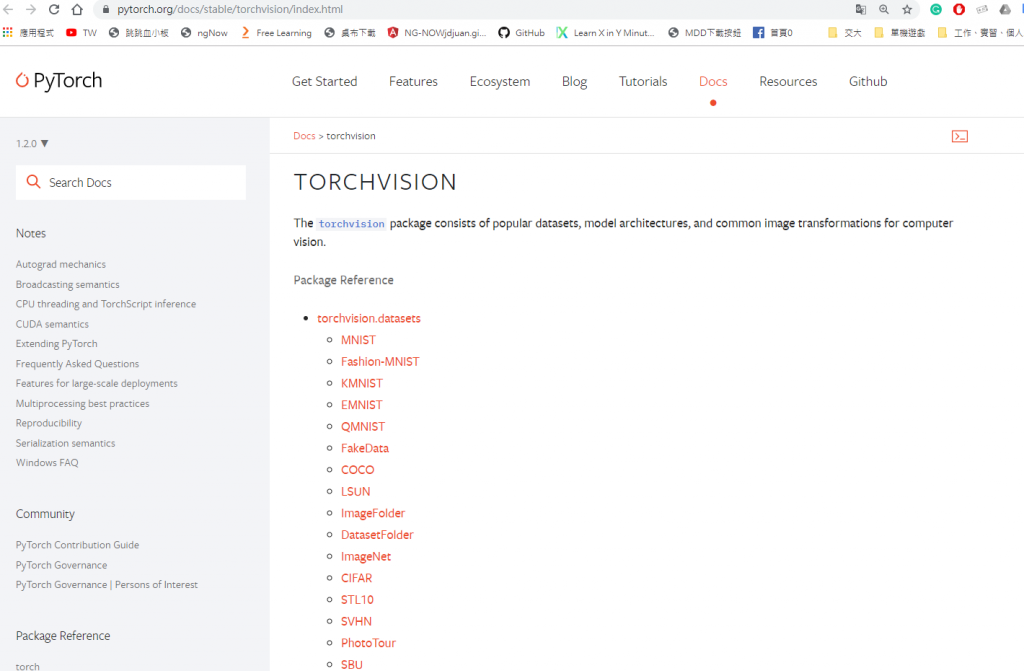Torchvision 是一個 Pytorch 官方維護的資源庫，裡面主要收錄三大類資源:

1. 有名、常用的 Dataset
• 像是 cifar-10 、 COCO 等等
2. 常見的 Image transform 工具
3. 有名、常用的 Model Architecture
• 例如 Alexnet 、 ResNe2 等等
利用 Torchvision 這個資源庫，我們可以快速使用不需自行處理的常用dataset、使用方便有效的image transform函式對我們的dataset做處理以及使用已經Pretrain 好的 model 等等。
安裝的方法可以很簡單，像是之前安裝 Pytorch 一樣:
``````pip install torchvision
``````

## 基本使用

1. 引入 (import) Torchvision
2. 使用 dataset
3. 使用 Transform
4. 使用 Model

### 使用/引入 Torchvision 資源庫

``````import torch
import torchvision
``````

``````torch.__version__
torchvision.__version__
``````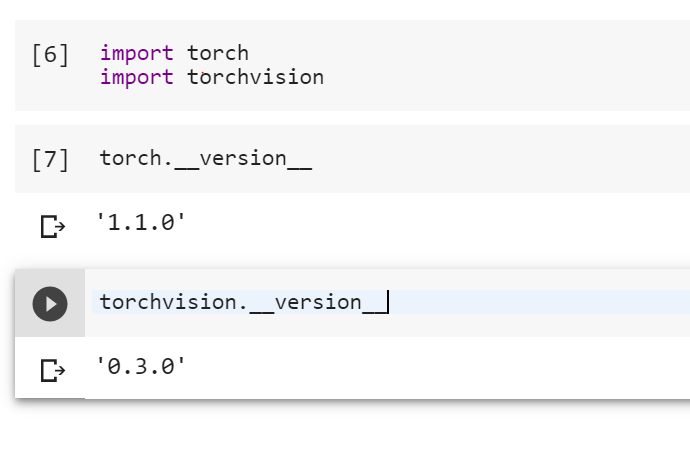### 使用Dataset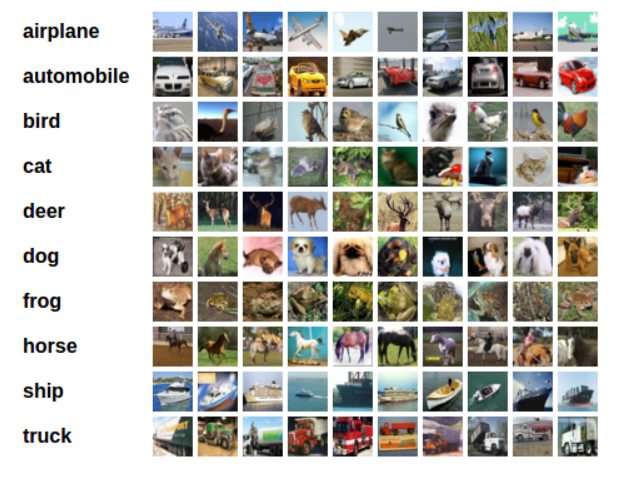0. Import 必須資料庫

``````import torch
import torchvision
import torchvision.transforms as transforms
``````

1.使用下面指令可以下載Cifar 10 的Training dataset 以及 Testing dataset

``````trainset = torchvision.datasets.CIFAR10(root='./data', train=True, download=True, transform=transform)
``````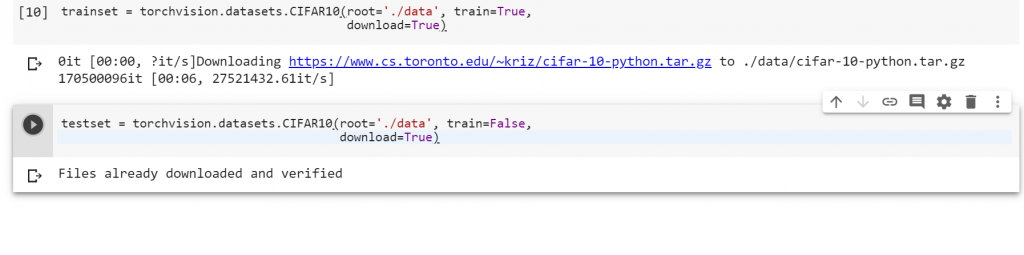2.使用 Dataloder 讀進這些 dataset 並加上 label
Dataloder是用來讀這些dataset的工具，目前我們先使用 pytorch 本身的dataloder即可，我們若有空會在實務篇講解一下dataloder內部細節以及如何自訂義 dataloder來讀自己的dataset

``````transform = transforms.Compose(
[transforms.ToTensor()])
``````

``````trainloader = torch.utils.data.DataLoader(trainset, batch_size=4,
shuffle=True, num_workers=2)
shuffle=False, num_workers=2)
``````

``````classes = ('plane', 'car', 'bird', 'cat',
'deer', 'dog', 'frog', 'horse', 'ship', 'truck')
``````

3.我們讓dataset 顯示

``````import matplotlib.pyplot as plt
import numpy as np

# functions to show an image

def imshow(img):
img = img / 2 + 0.5     # unnormalize
npimg = img.numpy()
plt.imshow(np.transpose(npimg, (1, 2, 0)))
plt.show()

# get some random training images
images, labels = dataiter.next()

# show images
imshow(torchvision.utils.make_grid(images))
print(' '.join('%5s' % classes[labels[j]] for j in range(4)))
``````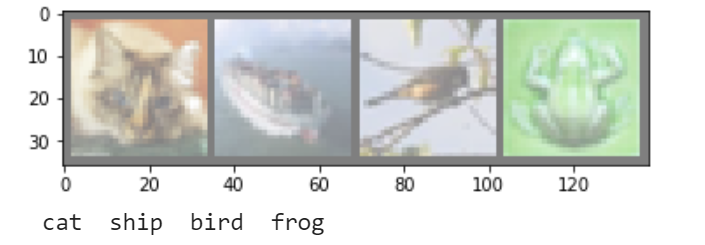### 使用 Transform

``````transform = transforms.Compose(
[transforms.ToTensor(),
transform.Translation(),
transforms.RandomHorizontalFlip(p=0.5)
])
``````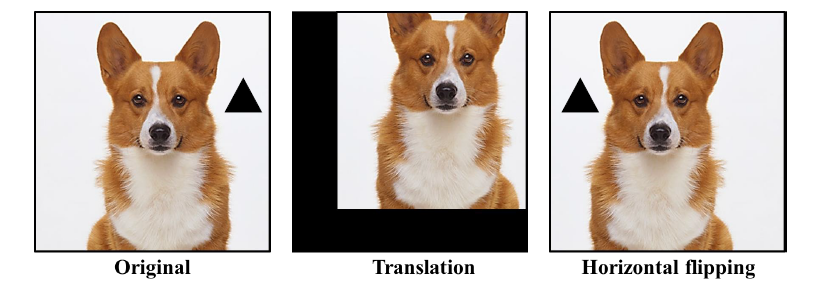### 導入 Pretrain Model

1.導入 torchvision 中的 models

``````import torchvision.models as models
``````

2.雕選自己想要的 Pretrain model，這裡以 Renet 18 當例子:

``````resnet18 = models.resnet18(pretrained = True)
``````

3.印出來看看是否成功導入model

``````print(resnet18)
``````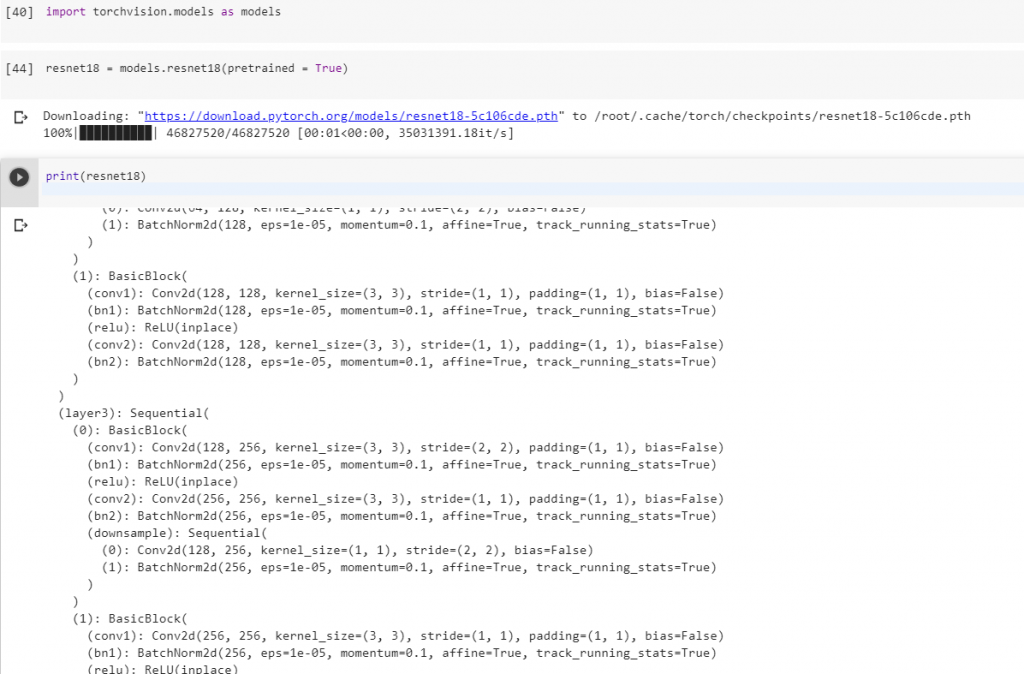# 結語

Torchvision 是一個十分好用且資源豐富的資源庫，日後可以各種多方嘗試看看各個功能!!